# PartialTwoPortFlow

Base class for distributed flow models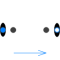# Information

This information is part of the Modelica Standard Library maintained by the Modelica Association.

Base class for distributed flow models. The total volume is split into nNodes segments along the flow path. The default value is nNodes=2.

#### Mass and Energy balances

The mass and energy balances are inherited from Interfaces.PartialDistributedVolume. One total mass and one energy balance is formed across each segment according to the finite volume approach. Substance mass balances are added if the medium contains more than one component.

An extending model needs to define the geometry and the difference in heights between the flow segments (static head). Moreover it needs to define two vectors of source terms for the distributed energy balance:

• `Qb_flows[nNodes]`, the heat flow source terms, e.g., conductive heat flows across segment boundaries, and
• `Wb_flows[nNodes]`, the work source terms.

#### Momentum balance

The momentum balance is determined by the `FlowModel` component, which can be replaced with any model extended from BaseClasses.FlowModels.PartialStaggeredFlowModel. The default setting is DetailedPipeFlow.

This considers

• pressure drop due to friction and other dissipative losses, and
• gravity effects for non-horizontal devices.
• variation of flow velocity along the flow path, which occur due to changes in the cross sectional area or the fluid density, provided that `flowModel.use_Ib_flows` is true.

#### Model Structure

The momentum balances are formulated across the segment boundaries along the flow path according to the staggered grid approach. The configurable `modelStructure` determines the formulation of the boundary conditions at `port_a` and `port_b`. The options include (default: av_vb):

• `av_vb`: Symmetric setting with nNodes-1 momentum balances between nNodes flow segments. The ports `port_a` and `port_b` expose the first and the last thermodynamic state, respectively. Connecting two or more flow devices therefore may result in high-index DAEs for the pressures of connected flow segments.
• `a_v_b`: Alternative symmetric setting with nNodes+1 momentum balances across nNodes flow segments. Half momentum balances are placed between `port_a` and the first flow segment as well as between the last flow segment and `port_b`. Connecting two or more flow devices therefore results in algebraic pressures at the ports. The specification of good start values for the port pressures is essential for the solution of large nonlinear equation systems.
• `av_b`: Asymmetric setting with nNodes momentum balances, one between nth volume and `port_b`, potential pressure state at `port_a`
• `a_vb`: Asymmetric setting with nNodes momentum balance, one between first volume and `port_a`, potential pressure state at `port_b`

When connecting two components, e.g., two pipes, the momentum balance across the connection point reduces to

`pipe1.port_b.p = pipe2.port_a.p`

This is only true if the flow velocity remains the same on each side of the connection. Consider using a fitting for any significant change in diameter or fluid density, if the resulting effects, such as change in kinetic energy, cannot be neglected. This also allows for taking into account friction losses with respect to the actual geometry of the connection point.

# Parameters (30)

allowFlowReversal Value: system.allowFlowReversal Type: Boolean Description: = true to allow flow reversal, false restricts to design direction (port_a -> port_b) Value: nNodes Type: Integer Description: Number of discrete volumes Value: system.energyDynamics Type: Dynamics Description: Formulation of energy balances Value: system.massDynamics Type: Dynamics Description: Formulation of mass balances Value: massDynamics Type: Dynamics Description: Formulation of substance balances Value: massDynamics Type: Dynamics Description: Formulation of trace substance balances Value: system.p_start Type: AbsolutePressure (Pa) Description: Start value of pressure at port a Value: p_a_start Type: AbsolutePressure (Pa) Description: Start value of pressure at port b Value: if n > 1 then linspace(p_a_start, p_b_start, n) else {(p_a_start + p_b_start) / 2} Type: AbsolutePressure[n] (Pa) Description: Start value of pressure Value: true Type: Boolean Description: Use T_start if true, otherwise h_start Value: if use_T_start then system.T_start else Medium.temperature_phX((p_a_start + p_b_start) / 2, h_start, X_start) Type: Temperature (K) Description: Start value of temperature Value: if use_T_start then Medium.specificEnthalpy_pTX((p_a_start + p_b_start) / 2, T_start, X_start) else Medium.h_default Type: SpecificEnthalpy (J/kg) Description: Start value of specific enthalpy Value: Medium.X_default Type: MassFraction[Medium.nX] (kg/kg) Description: Start value of mass fractions m_i/m Value: Medium.C_default Description: Start value of trace substances Value: 1 Type: Real Description: Number of identical parallel flow devices Value: Type: Length[n] (m) Description: lengths of flow segments Value: Type: Area[n] (m²) Description: cross flow areas of flow segments Value: Type: Length[n] (m) Description: hydraulic diameters of flow segments Value: Type: Roughness[n] (m) Description: Average heights of surface asperities Value: zeros(n) Type: Length[n] (m) Description: Differences in heights of flow segments Value: system.momentumDynamics Type: Dynamics Description: Formulation of momentum balances Value: system.m_flow_start Type: MassFlowRate (kg/s) Description: Start value for mass flow rate Value: 2 Type: Integer Description: Number of discrete flow volumes Value: Types.ModelStructure.av_vb Type: ModelStructure Description: Determines whether flow or volume models are present at the ports Value: false Type: Boolean Description: =true to lump pressure states together Value: if useLumpedPressure then nFMLumped else nFMDistributed Type: Integer Description: number of flow models in flowModel Value: if modelStructure == Types.ModelStructure.a_v_b then n + 1 else if modelStructure == Types.ModelStructure.a_vb or modelStructure == Types.ModelStructure.av_b then n else n - 1 Type: Integer Value: if modelStructure == Types.ModelStructure.a_v_b then 2 else 1 Type: Integer Value: integer(n / 2) + 1 Type: Integer Description: Index of control volume with representative state if useLumpedPressure Value: false Type: Boolean Description: =true to take port properties for flow models from internal control volumes

# Inputs (1)

fluidVolumes Default Value: array(crossAreas[i] * lengths[i] for i in 1:n) * nParallel Type: Volume[n] (m³) Description: Discretized volume, determine in inheriting class

# Connectors (2)

port_a port_b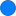Type: FluidPort_a Description: Fluid connector a (positive design flow direction is from port_a to port_b)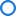Type: FluidPort_b Description: Fluid connector b (positive design flow direction is from port_a to port_b)

# Components (6)

system mediums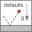Type: System Description: System properties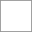Type: BaseProperties[n]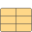Type: ThermodynamicState Description: state defined by volume outside port_aType: ThermodynamicState Description: state defined by volume outside port_bDescription: state vector for flowModel model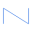Type: FlowModel Description: Flow model

# Extended by (1)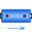DynamicPipe Modelica.Fluid.Pipes Dynamic pipe model with storage of mass and energy